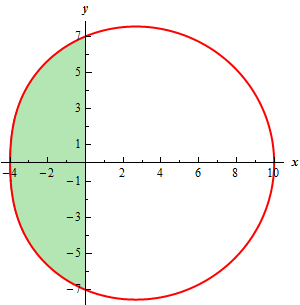Paul's Online Notes
Home / Calculus II / Parametric Equations and Polar Coordinates / Area with Polar Coordinates
Show Mobile Notice Show All Notes Hide All Notes
Mobile Notice
You appear to be on a device with a "narrow" screen width (i.e. you are probably on a mobile phone). Due to the nature of the mathematics on this site it is best views in landscape mode. If your device is not in landscape mode many of the equations will run off the side of your device (should be able to scroll to see them) and some of the menu items will be cut off due to the narrow screen width.

### Section 9.8 : Area with Polar Coordinates

2. Find the area inside the graph of $$r = 7 + 3\cos \theta$$ and to the left of the $$y$$-axis.

Show All Steps Hide All Steps

Start Solution

First, here is a quick sketch of the graph of the region we are interested in.Show Step 2

For this problem there isn’t too much difficulty in getting the limits for the problem. We will need to use the limits $$\frac{\pi }{2} \le \theta \le \frac{{3\pi }}{2}$$ to trace out the portion of the graph to the left of the $$y$$-axis.

Remember that it is important to trace out the portion of the curve defining the area we are interested in as the $$\theta$$’s increase from the smaller to larger value.

Show Step 3

The area is then,

\begin{align*}A & = \int_{{\frac{\pi }{2}}}^{{\frac{{3\pi }}{2}}}{{\frac{1}{2}{{\left( {7 + 3\cos \theta } \right)}^2}\,d\theta }}\\ & = \frac{1}{2}\int_{{\frac{\pi }{2}}}^{{\frac{{3\pi }}{2}}}{{49 + 42\cos \theta + 9co{s^2}\left( \theta \right)\,d\theta }}\\ & = \frac{1}{2}\int_{{\frac{\pi }{2}}}^{{\frac{{3\pi }}{2}}}{{49 + 42\cos \theta + \frac{9}{2}\left( {1 + \cos \left( {2\theta } \right)} \right)\,d\theta }}\\ & = \frac{1}{2}\int_{{\frac{\pi }{2}}}^{{\frac{{3\pi }}{2}}}{{\frac{{107}}{2} + 42\cos \theta + \frac{9}{2}\cos \left( {2\theta } \right)\,d\theta }}\\ & = \left. {\frac{1}{2}\left( {\frac{{107}}{2}\theta + 42\sin \left( \theta \right) + \frac{9}{4}\sin \left( {2\theta } \right)} \right)} \right|_{\frac{\pi }{2}}^{\frac{{3\pi }}{2}} = \require{bbox} \bbox[2pt,border:1px solid black]{{42.0376}}\end{align*}

Make sure you can do the trig manipulations required to do these integrals. Most of the integrals in this section will involve this kind of manipulation. If you don’t recall how to do them go back and take a look at the Integrals Involving Trig Functions section.